≡ Menu

Advertisement

Advertisement

# Review of Tesla Capital Structure and Leverage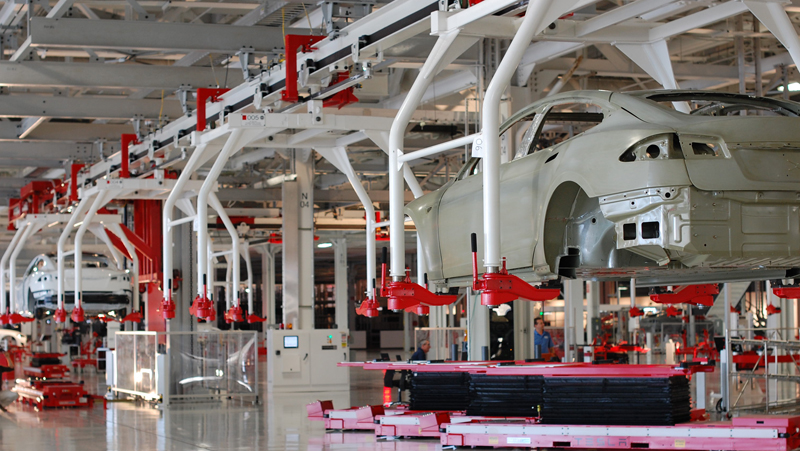A Tesla factory in Fremont, CA. Source: Flickr

Tesla (NASDAQ: TSLA) debt to equity ratio is an important metric that tells about the capital structure of the company.

From the ratio, investors and analysts can find out how Tesla funded its balance sheets, whether by debts or by equity or both.

Ideally, the capital structure of a company should be well balanced between debts and equity.

For example, for every 1 dollar of equity, there is 1 dollar of debt. In this case, the debt to equity ratio will be 1.

However, in real life, this balanced debt structure scenario is not always the case for most companies.

The reason is that it may not be prudent to rely largely on equity finances.

In most cases, the cost of debt may be much cheaper than the required rate of return on equity.

This is particularly true in a low-interest-rate environment.

In this article, we will explore Tesla’s capital structure to find out how the company leverages using debts and equity.

Let’s get started!

## Equation for Debt to Equity Ratio

Before illustrating further, the equation that I used to calculate the debt to equity ratio is shown as below:

Debt to Equity Ratio = Debt / Equity

From the above equation, the equity part is easily understood.

Basically, it’s the total equity or the book value that can be easily obtained from the balance sheets.

## Tesla’s Equities

Tesla’s equities include both shareholders’ equity and minority interest equity.

Two types of equities exist because Tesla is a holding company of multiple subsidiaries.

As such, the balance sheet is consolidated with the assets and liabilities of all subsidiaries.

The consolidation has led to the existence of minority interests in the balance sheet since Tesla does not own 100% of some subsidiaries.

The following snapshot illustrates Tesla’s non-controlling interest or minority interest portion in the balance sheet.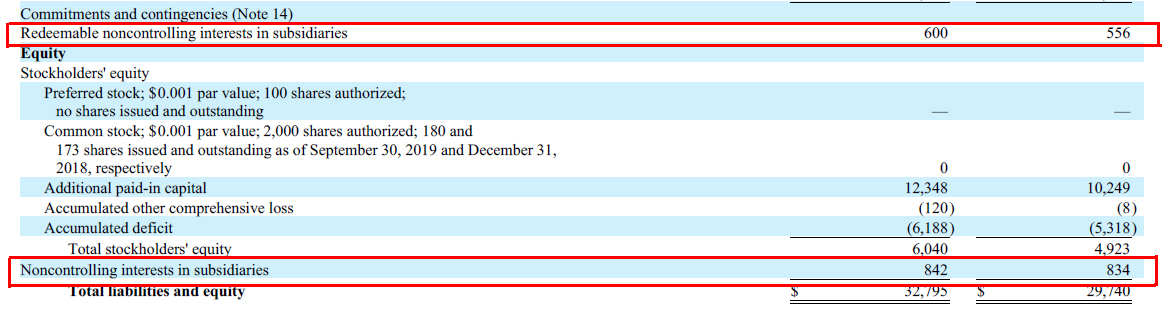Tesla minority interest

Tesla’s minority interest exists as shown in the balance sheet above is due mainly to the company’s ownership structure.

## Tesla’s Liabilities

Coming back to the debt to equity equation above, difficulties may arise when we try to figure out the debt part of the equation.

There are countless definitions of “debts”. In this case, debts can be derived from long-term or current debts, total liabilities or interest-bearing only debts.

For simplicity, I have considered only “debts” that matters most in the analysis of Tesla’s leverages.

Here are some of the most commonly used interpretations of debts which will be further illustrated in subsequent discussion:

1. Total debts – debt instruments that include both long and short-term loans as well as finance and operating leases. Basically, total debts are all interest-bearing debt instruments.

2. Long-term liabilities – these are long-term loans and other miscellaneous liabilities such as accounts payable, deferred revenues, leases, warranty reserves and so on. Only the long-term portion is measured in this case.

3. Total liabilities – these are all the liabilities in the balance sheet that are comprised of both long and short-term loans as well as other long and short liabilities such as accounts payable, deferred revenues and other miscellaneous liabilities.

The subsequent debt to equity ratio discussion will revolve around these 3 types of “debts” definitions mentioned above.

## Tesla’s Total Debt

Let’s first briefly look at Tesla’s total debt.

As briefly mentioned in the prior discussion, Tesla’s total debt consists of mainly interest-bearing debt instruments, including long-term and current loans.

In this discussion, the total debt includes both finance and operating leases as these are also interest-bearing debt instruments which have added a substantial amount of debt to the company as detailed in this article: Tesla debts analysis.

The leases, both operating and finance, represent the total minimum lease payment which also includes the expected interest portion in the future.

The following snapshot shows an example of the total minimum lease payment disclosed in one of Tesla’s financial statement: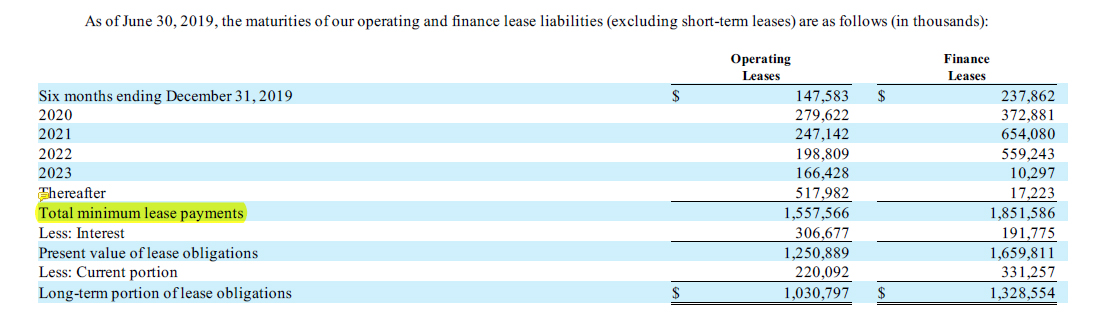Tesla operating and finance leases from the quarterly statement

Other liabilities, including accounts payable, accrued liabilities, deferred revenue, warranty reserves and so on are excluded in the calculation of Tesla’s total debt.

In short, Tesla’s total debt consists of only interest-bearing debt instruments and leases, both finance and operating.

## Tesla’s Total Debts to Equity Ratio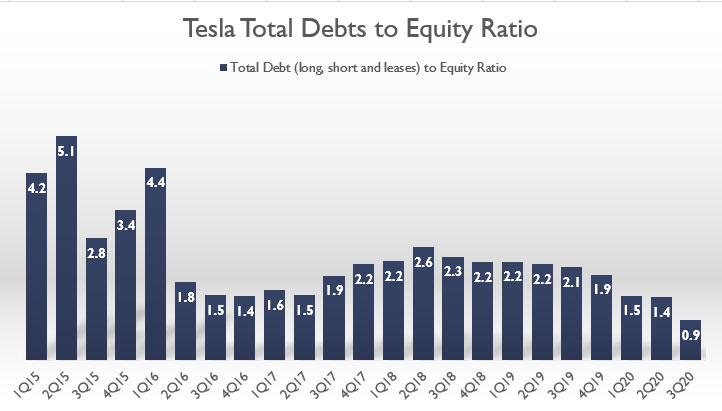Tesla total debt to equity ratio

The chart above shows Tesla’s total debts to equity ratio over the last 5 years.

As mentioned, the plot above illustrates Tesla’s leverage in using only interest-bearing debts with respect to equity.

As seen from the chart, Tesla’s total debt to equity ratio was the highest back in 2015 when the total debt was averagely 4 times more than that of equity.

However, the ratio dropped substantially to only 1.8 in 2Q 2016 from its previous high of 4.4.

Upon investigation, Tesla made a very huge equity issuance for capital raise in 2Q 2016 which had significantly increased the company’s equity.

For this reason, Tesla’s total debts to equity ratio decreased more than double to only 1.8 in 2Q 2016 from the prior quarter.

Since 2016, Tesla’s debt to equity ratio further declined to a record low of 1.5 in 2017 but the ratio slightly bounced back to 2.0 in 2018.

The debt to equity ratio stayed at around the same level at 2.0 since 2018.

Again, Tesla issued 3 million shares in March 2020 to raise \$2.31 billion of cash, resulting in a substantial rise in the company’s equity in Q1 2020.

As a result, Tesla’s debt to equity ratio decreased further to only 1.5 in 2020 1Q.

As of 2020 3Q, Tesla’s debt to equity ratio stood at only 0.9, the lowest in the last 5 years.

At a ratio of 0.9, Tesla’s total debt leverage with respect to equity was very low in Q3 2020.

In fact, at this ratio, Tesla has about \$0.90 dollar of interest-bearing debt for every \$1.00 dollar of equity.

There are a few reasons for the ratio to decline steadily and reached the lowest at 0.9 as of 2020 3Q.

One of the likely reasons can be attributed to the fact that Tesla has borrowed less debt but issued more equity between 2018 and 2020.

As you may have already known, equity is less risky compared to debt borrowings because Tesla literally does not have to repay the capital obtained through equity.

The only downside to equity is that it dilutes the control of the company.

## Tesla Long-Term Liabilities to Equity RatioTesla long-term liabilities to equity ratio

The above chart shows Tesla’s long-term liabilities to equity ratio between 2015 and 2020.

As mentioned, Tesla’s long-term liabilities consist of all non-current liabilities such as long-term loans, leases, accrued liabilities, deferred revenues, resale value guarantee and other miscellaneous long-term liabilities.

As shown in the chart above, Tesla’s long-term liabilities to equity ratio plot looks very similar to the total debts to equity ratio plot shown in the earlier discussion.

In fact, the trend of the current plot looks exactly the same as the prior chart.

For instance, Tesla was highly leveraged back in 2015 when the long-term liabilities to equity ratio was averaged around 3.8.

The ratio significantly declined in 2016 but had slightly ticked up during 2017 to nearly 3.0.

Since then, the ratio declined steadily and reached the lowest figure at only 0.8 as of Q3 2020.

Tesla was minimally leveraged when the long-term liabilities to equity ratio was at 0.8, meaning that Tesla has about \$0.80 dollar of long-term liabilities to \$1.00 of equity.

Similarly, the decline of the ratio has been largely attributed to the increasing issuance of equity between 2018 and 2020 considering that equity issuance is less risky compared to debt offerings.

Besides, it’s also to Tesla’s advantage when it issued equity at a time when the company was valued at more than \$500 billion.

In this case, Tesla can issue fewer equities to obtain a larger capital.

## Tesla Total Liabilities to Equity Ratio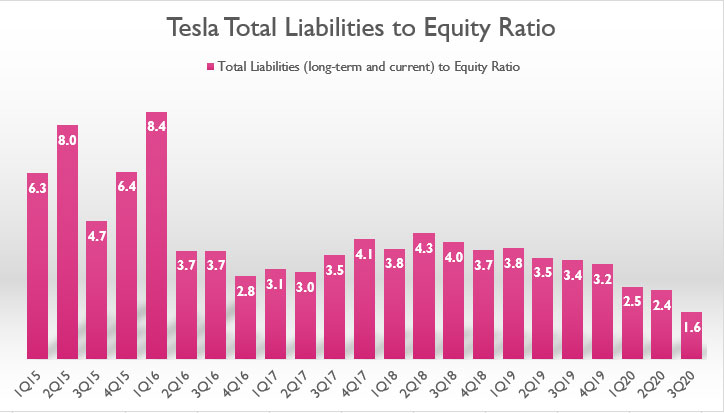Tesla total liabilities to equity ratio

The chart above shows Tesla’s total liabilities to equity ratio over the past 5 years from 2015 to 2020.

Tesla’s total liabilities are basically all long and short-term borrowings and whatever the company owes to 3rd parties in the balance sheets.

In terms of total liabilities, the trend of Tesla’s total liabilities to equity ratio also looks similar to prior charts.

The only difference is that the leverage ratio has been much higher in the current chart.

This is expected as all liabilities are included in the measurement of the debt to equity ratio.

Again, a similar trend is observed when the ratio slowly declined from its peak of roughly 4.3 recorded in 2Q 2018 to only 1.6 in 3Q 2020.

Tesla’s total liabilities to an equity leverage ratio of 1.6 reported in Q3 2020 was the lowest over the past 5 years.

In other words, Tesla has the lowest leverage between total liabilities and equity as of Q3 2020 despite a record amount of debt in the same quarter.

This scenario is expected when the company’s valuation has been rising steadily in the last 5 years.

As mentioned, it is to Tesla’s advantage to obtain capital through equity issuance since the company can issue less equity but is able to get a much larger capital.

## Total Liabilities to Total Funds (Total Assets) Ratio

Finally, this is probably the most important ratio among all other ratios that have been discussed.

The total liabilities to total funds ratio measures the company leverage in terms of total liabilities over total funds or total assets.

Total funds are the total assets of the company.

Basically, total funds are the sum of total liabilities and shareholders’ equity which equals the total assets of the company.

Here is the equation for the calculation of this ratio:

Total Liabilities to Total Funds Ratio = [ Total Liabilities / Total Assets ] X 100%

The above ratio, expressed in percentage, measures the percentage of the balance sheet funded by debt or liabilities.

Instantly, it gives an overview of the funding side of the balance sheet, whether entirely from debts or from equity.

In other words, the ratio tells us about the proportion of the total assets that are funded by debts and equity.

In short, the total liabilities to total funds ratio reflects the capital structure of a company.

Let’s take a look at Tesla’s capital structure in the following chart.

## Tesla’s Capital Structure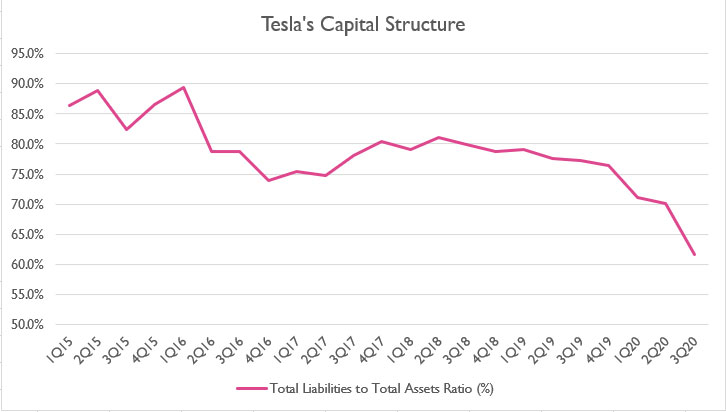Tesla capital structure

The chart above clearly shows that Tesla’s capital structure has been entirely funded by debt or liabilities as the numbers have been on the high side in the last 5 years.

Throughout the shown period from 2015 to 2020, the ratio has been consistently above 70%, with some ratios even exceeding 90% back in 2015.

This ratio says that as much as 90% of Tesla’s capital structure was funded by liabilities back in 2015.

However, Tesla’s total liabilities to total funds ratio has been declining since 2015.

Between 2016 and 2018, Tesla’s capital structure was still pretty much made up entirely of liabilities.

Albeit slightly lower, the ratio was still around 80%, indicating that the company raised much of its capital thru debts.

From 2019 and onward, Tesla’s capital structure has slowly headed towards equity funding instead of using debt, as seen from the declining total liabilities to total assets ratio.

As of 2020 3Q, Tesla’s total liabilities to total funds ratio has dropped to its lowest level at around 60%, illustrating a steady structural change in the company’s capital over the last 5 years.

The result in the chart above is in line with prior debt to equity ratios that we have seen where they have all reached all-time lows as of 2020 Q3.

While there have been changes in Tesla’s capital structure, its balance sheet was still 60% liabilities and 40% equity as of 3Q 2020, indicating that Tesla still relies mainly on debt as its main source of capital.

At this composition, Tesla’s leverage was moderately low at around \$1.60 dollars of liabilities to \$1.00 dollars of equity in 2020.

## Tesla’s Debt to Asset Ratio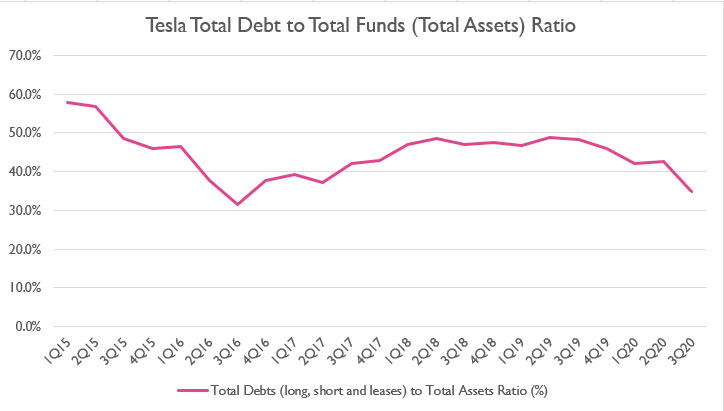Tesla total debt to assets ratio

When we looked at Tesla’s total debt as opposed to total liabilities, the chart above shows that Tesla’s debt ratio was even lower against the company’s total assets.

As of 2020 Q3, Tesla’s debt ratio was around 35% of total assets, suggesting that the company carried only \$0.35 dollars of interest-bearing debt out of \$1.00 dollars of assets.

The figure was at an all-time low since 2017.

Do note that Tesla’s total debt to total assets ratio has actually increased slightly between 2017 and 2019 before heading lower again as of 2020.

The uptrend during 2018 suggests that Tesla has actually borrowed more debt than equity to fund its capital requirement.

However, the downtrend in 2020 suggests that Tesla has slowly avoided debt and preferred equity as its main sources of funding.

## Conclusion

To recap, Tesla’s total debts to equity ratio has significantly declined from its peak value of more than 4.0 in 2015 to only 0.9 as of 2020 Q3.

The downtrend is also observed for the total liabilities to equity ratio, indicating that Tesla’s preference for funding has shifted towards equity over debt.

The trend is understandable when Tesla’s valuation is slowly gaining ground and has since surpassed more than \$500 billion by 4Q 2020.

At this enormous valuation, Tesla can obtain a higher capital through equity issuance even though this practice may mean further dilution to the existing stockholders.

## References and Credits

1. Financial figures in all charts above were obtained and referenced from financial statements available in Tesla Quarterly Results.

2. Featured image was used under Creative Common Licenses and obtained from mangopulp2008 and Maurizio Pesce.

## Disclosure

Readers, investors, analysts, bloggers, visitors, researchers, writers, or academicians are highly encouraged to use, copy, quote, distribute, duplicate, modify, edit, upload, download, share and link any materials on this webpage such as the charts, snapshots, texts, paragraphs, etc. You can credit back to this page by a link or a mention of the website. Thanks for sharing!

{ 2 comments… add one }
•Anna December 18, 2020, 7:21 am

Hello!
Just wondering how did you calculate total debt to equity ratio as I am slightly confused looking at tesla’s financial statements. Thank you!

•cckean December 18, 2020, 9:42 am

Just take the total debt and divide it over the total equity.

Cancel reply

Leave a Comment

X